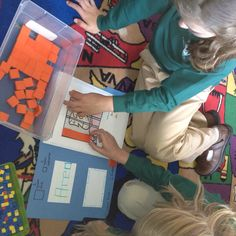Math Puzzles

# Puzzle time! Can you do these area and perimeter questions?

2.8k views

 1 Introduction 2 How to find perimeter? 3 Examples 4 Conclusion 5 About Cuemath 6 FAQ’s 7 External references:

11 November  2020

## Introduction

### What is area and perimeter?

Area: A part of the plane enclosed by a simple closed figure is called a plane region and the measurement of plane region enclosed is called its area. Area is measured in square units.

Perimeter: The length of the boundary of a closed figure is called the perimeter of the plane figure. The units of perimeter are the same as that of length, i.e., m, cm, mm, etc.

### Formulas:

Perimeter and Area of Rectangle:

● The perimeter of rectangle = 2(l + b).
● Area of rectangle = l × b; (l and b are the length and breadth of rectangle)

Perimeter and Area of Square:

● Perimeter of square = 4 × S.
● Area of square = S × S.

Also, try:

 📥 Puzzle time! Can you do these area and perimeter questions? Download

## How to find perimeter?

We utilize a ruler to find the length of the sides of a little standard shape. The border is controlled by adding the lengths of the sides/edges of the shape.

For little unpredictable shapes, we can utilize a line of string and spot it precisely along the limit of the shape, once. The complete length of the string utilized along the limit is the edge of the shape.## Examples### Perimeter of square:

 Example 1

Find the perimeter of a square shaped playground whose each side length is 12 cm.

### Solution

We know a square has four equal sides. So, we can easily calculate the perimeter of a square.

The formula for finding the perimeter of a square is:

P = 4 × length of a side

= 4 × 12 cm

 = 48 cm

### Perimeter of rectangle

 Example 1

A rectangular yard has length equals to 12 cm and breadth equals to 15 cm. Find its perimeter.

### Solution

Length = 12 cm and Breadth = 15 cm

We know,

The perimeter of a rectangle = 2(length + width)

Substitute the value of length and width here,

Perimeter, P = 2(12 + 15) cm

P = 2 x 27 cm

 Therefore, the perimeter of a rectangle = 54 cm

### Area of rectangle

 Example 1

Find the area of a rectangular blackboard whose length and breadth are 130 cm and 90 cm, respectively.

### Solution

Length of the blackboard = 130 cm

Breadth of the blackboard = 90 cm

Area of the blackboard = area of a rectangle = length x breadth = 130 cm x 90 cm

 = 1170 cm

### Area of square

 Example 1

Find the area of the square of side 16 cm.

### Solution

Side of the square = a = 16 cm

Area of the square = a2

= 162 cm2

 = 256 cm2

Also, try:

## Conclusion

The first step you can take is to keep the formula in mind here are the few tips that will help you

• Practice concentration
• Practice as much as you can
• Understand the basic concept of the formula
• Use memorising tricks

If you know this particular concept it will be useful in real life also, For example :

• Fencing off an area to plot a crop.
• Planning the construction of a house.
• Building a barn with box stalls for horses.
•  Building a swimming pool.
• Wood designs.

Hopefully you now see how important area and perimeter is. It is how you stay sheltered!  Your house is shaped and sized the way it is because of the usage of area and perimeter.

These two concepts touch everything; including our houses, cars, electronics we all use, and even the furniture we sit/lay on!  Believe it or not, every building you see has a documented area and/or perimeter!  If you've got a tape-measure at home (if you don't I can provide one for you), I want you to find both the area and perimeter of your house.  If that is too much, then find the area/perimeter of your own room.

You could conclude by saying that finding the perimeter and area of a square and rectangle of a given question also helps in your real life. Cuemath explains these kinds of problems by taking real examples so that it helps you to remember and understand for a longer period of time.

Cuemath, a student-friendly mathematics platform, conducts regular Online Live Classes for academics and skill-development and their Mental Math App, on both iOS and Android, is a one-stop solution for kids to develop multiple skills.Understand the Cuemath Fee structure and sign up for a free trial.

## How to find the Perimeter of a Square?

To calculate the perimeter of a square, add all the sides of it. There are 4 sides of a square which are all equal in length. The sum of the sides will give its perimeter.

## How to find the perimeter of a rectangle?

To get the perimeter of a rectangle, add all the sides of it. There are 4 sides of a rectangle whose sum will give its perimeter.

## External references:

### Area of Circle, Triangle, Square, Rectangle, Parallelogram …

Related Articles
Award-winning math curriculum, FREE for a year